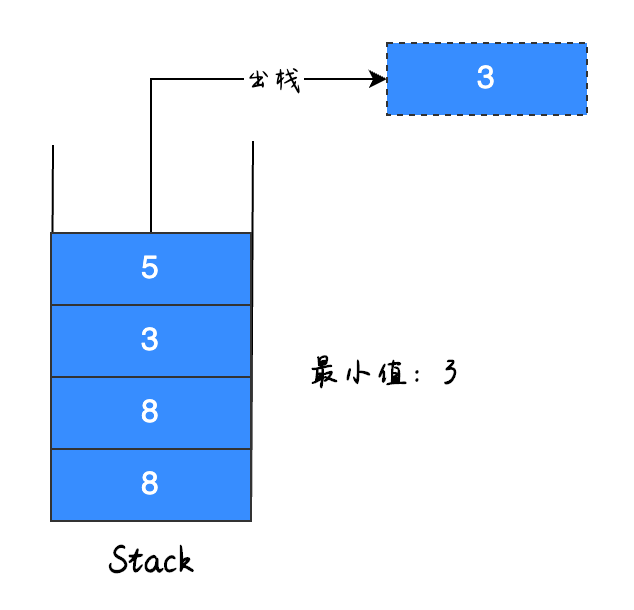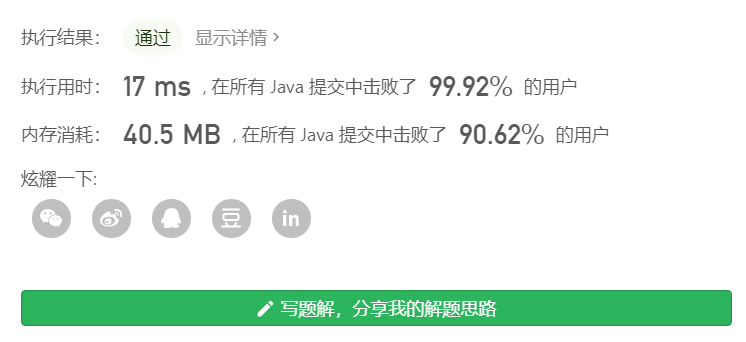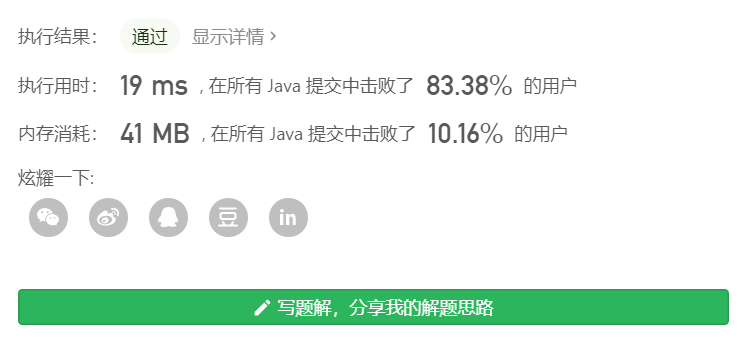### 题目

MinStack minStack = new MinStack();

minStack.push(-2);

minStack.push(0);

minStack.push(-3);

minStack.min();   --> 返回 -3.

minStack.pop();

minStack.top();      --> 返回 0.

minStack.min();   --> 返回 -2.

LeetCode 地址：leetcode-cn.com/problems/ba…

### 思考

• 当我们进行 pop（移除栈顶元素）操作时如果删除的是当前最小值，那么我们如何寻找下一个最小值？
• 要保证调用 min、push 及 pop 的时间复杂度都是 O(1)。

### 解题思路

#### 操作步骤5### 实现代码1

``````class MinStack {
private int[] data; // 栈数据
private int maxSize; // 最大长度
private int top; // 栈顶指针（下标）
private int min; // 最小值

// 构造函数
public MinStack() {
// 设置默认值
maxSize = 10000;
data = new int[maxSize];
top = -1;
min = Integer.MAX_VALUE;
}

// 入栈（添加元素）
public void push(int x) {
if (min >= x) {
// 遇到了更小的值，记录原最小值（入栈）
data[++top] = min;
min = x;
}
// 当前值入栈
data[++top] = x;
}

// 出栈（移除栈顶元素）
public void pop() {
if (min == data[top]) {
min = data[--top]; // 拿到原最小值，并（将原最小值）出栈
}
--top; // 出栈
}

// 查找栈顶元素
public int top() {
return data[top];
}

// 查询最小值
public int min() {
return min;
}
}### 实现代码2

``````class MinStack {
private Stack<Integer> stack = new Stack<>();
private int min = Integer.MAX_VALUE;

public MinStack() { }

// 入栈（添加元素）
public void push(int x) {
if (x <= min) {
// 遇到了更小的值，记录原最小值（入栈）
stack.push(min);
min = x;
}
stack.push(x);
}

// 出栈（移除栈顶元素）
public void pop() {
if (stack.pop() == min) {
min = stack.pop(); // 取出原最小值
}
}

// 查找栈顶元素
public int top() {
return stack.peek();
}

// 查询最小值
public int min() {
return min;
}
}### 最后java

0